What is paraconsistent logic?

In this post will be explained what is paraconsistent logic, how to deal with non-trivial contradictions and your applications to robotics and automation.

Limits of classical logic

The classical logic only can determine if something is true or false. In many real situations, the answer can’t be given like “X” or “not X”, exist inconsistences, paradoxes, undefinitions and ambiguous answers. Machines which operate with classical logic have difficult to solve many real problems and are unable to give a proper answer.

Paraconsistent logic annotated

In paraconsistent logic annotated PLA2v, there are 2 values which represents the certainly and contraction degrees, in horizontal and vertical respectively. The classifications are: false (F), true (t), indeterminate (⊥) and inconsistent (T). Can assume values between 0 and 1.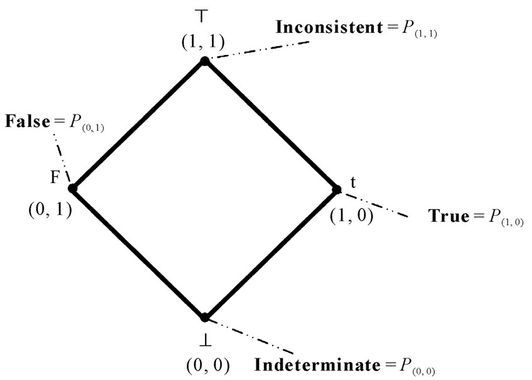From the diagram above, can obtain a graphic.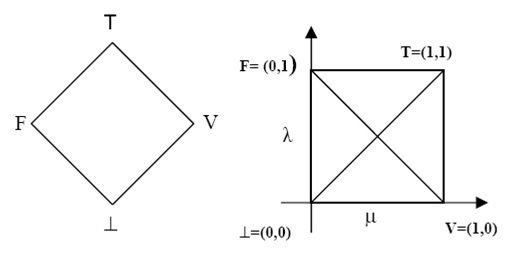This graphic can be divided in 12 regions, 4 extremes and 8 non-extremes.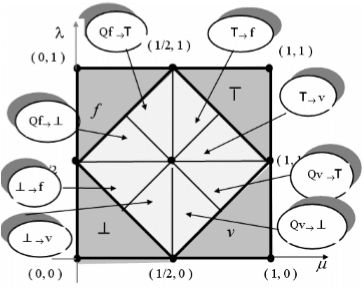Source: (Filho, 2006)

The Q means almost and the symbol → represents “tending to”. For example, T→v means “inconsistent tending to true” and Qf→⊥ means “almost false tending to indeterminate”. This is certainly degree formula $Dc$, can vary between -1 and 1. If equal to 1, it is totally true, if equal to -1, is totally false.

$Dc=\mu -\lambda$

This is the formula of uncertainly or contradiction degree $Dct$, also varies from -1 to 1. If it is equal to 1, is totally inconsistent, in case is -1, is totally indeterminate or paracomplete.

$Dct=\mu +\lambda-1$

A paraconsistent logic with three values (PLA3v), includes specialty degree e, which is an extra dimension in the graphic.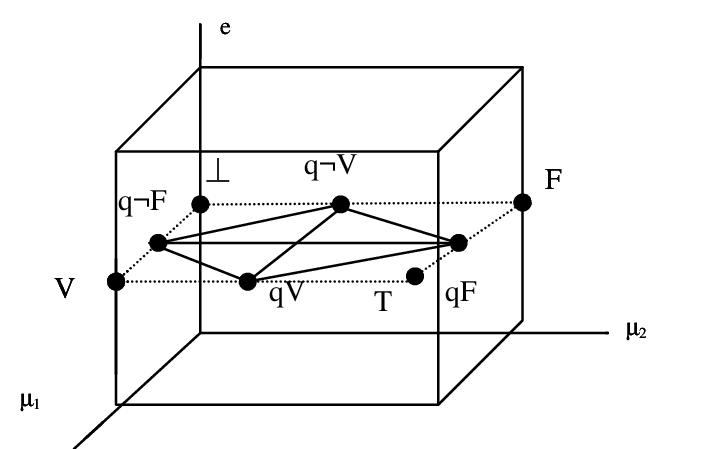With 4 values, it is included the temporality degree.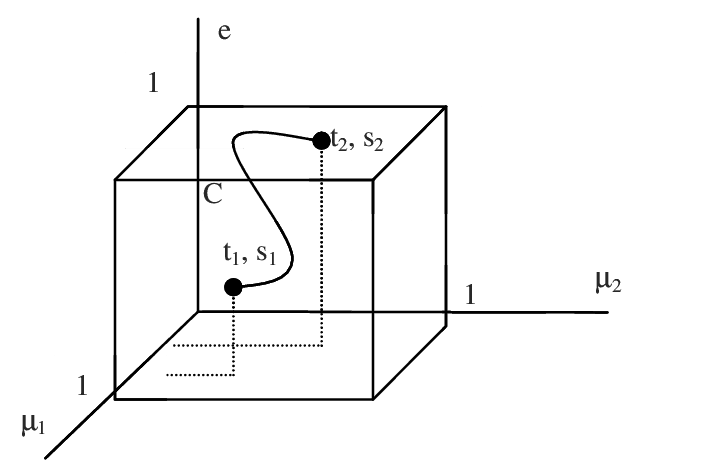Applying in robotics

A mobile robot moving in an environment must have many sensors to detect obstacles, avoid collisions and get as many information as possible about the environment. Many sensors will send contradictory signals which can`t have a adequate treatment with classical logic. Some sensor signals represent $\mu$ and $\lambda$. The certainly and uncertainly degrees are calculated by the controller and one of the 12 logic states is chosen as output. The robot follow the routine according with the logic state. This is Emmy, the first mobile robot with paraconsistent logic, built in 1999.Mobile autonomous robots with non-classical logic can be used in dangerous environment like radioactive areas, zones with poisonous gases, deep sea, other planets, space.